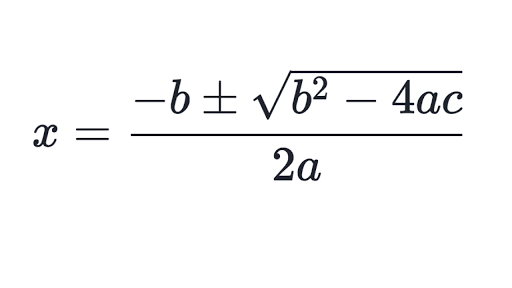Published: March 19, 2019

How to find the roots of..

x²+6x-9 = 0

..in Java?

That's what this post is about. We will be using quadratic formula.

```public static int[] getRoots(int a, int b, int c) { // ax²+bx+c=0
```

The output will be given as an array with the roots.

The quadratic formula looks like this:```public static int[] getRoots(int a, int b, int c) { // ax²+bx+c=0
double x1 = (-b + Math.sqrt( b * b - 4 * a * c ))/( 2 * a );
double x2 = (-b - Math.sqrt( b * b - 4 * a* c)) / ( 2 * a );
return new int [] { x1, x2 };
}
```

Of course there is a possibility of getting a negative number in the square root. In order to make sure this won't happen, you can do this:

```public static int[] getRoots(int a, int b, int c) { // ax²+bx+c=0
if((b * b - 4 * a * c) < 0)
return null; // No solution
double x1 = (-b + Math.sqrt( b * b - 4 * a * c ))/( 2 * a );
double x2 = (-b - Math.sqrt( b * b - 4 * a* c)) / ( 2 * a );
return new int [] { x1, x2 };
}
```

Of course if x1 and x2 is the same, you might as well only return one number

```public static int[] getRoots(int a, int b, int c) { // ax²+bx+c=0
if((b * b - 4 * a * c) < 0)
return null; // No solution
double x1 = (-b + Math.sqrt( b * b - 4 * a * c )) / ( 2 * a );
double x2 = (-b - Math.sqrt( b * b - 4 * a * c )) / ( 2 * a );
if(x1 == x2)
return new int [] { x1 };
return new int [] { x1, x2 };
}
```

You may also want to read...